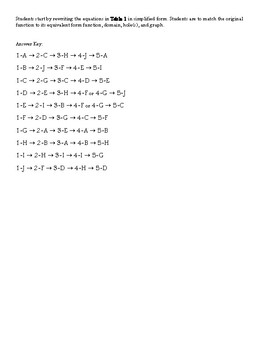# Rational Function Graphs With Holes Matching ActivitySubject
Resource Type
File Type

PDF

(445 KB|2 pages)
Product Rating
Standards
• Product Description
• StandardsNEW

This matching activity has a total of 10 different rational function problems. The goal is to match a given function to its equivalent form after it has been simplified. Then students can match the equivalent form of the function to its domain, location of holes, and the graph.

They should also know how to find the vertical and horizontal asymptotes of a rational function.

Understand that rational expressions form a system analogous to the rational numbers, closed under addition, subtraction, multiplication, and division by a nonzero rational expression; add, subtract, multiply, and divide rational expressions.
Rewrite simple rational expressions in different forms; write 𝘢(𝘹)/𝘣(𝘹) in the form 𝘲(𝘹) + 𝘳(𝘹)/𝘣(𝘹), where 𝘢(𝘹), 𝘣(𝘹), 𝘲(𝘹), and 𝘳(𝘹) are polynomials with the degree of 𝘳(𝘹) less than the degree of 𝘣(𝘹), using inspection, long division, or, for the more complicated examples, a computer algebra system.
Know and apply the Remainder Theorem: For a polynomial 𝘱(𝘹) and a number 𝘢, the remainder on division by 𝘹 – 𝘢 is 𝘱(𝘢), so 𝘱(𝘢) = 0 if and only if (𝘹 – 𝘢) is a factor of 𝘱(𝘹).
Total Pages
2 pages
Included
Teaching Duration
1 hour
Report this Resource to TpT
Reported resources will be reviewed by our team. Report this resource to let us know if this resource violates TpT’s content guidelines.